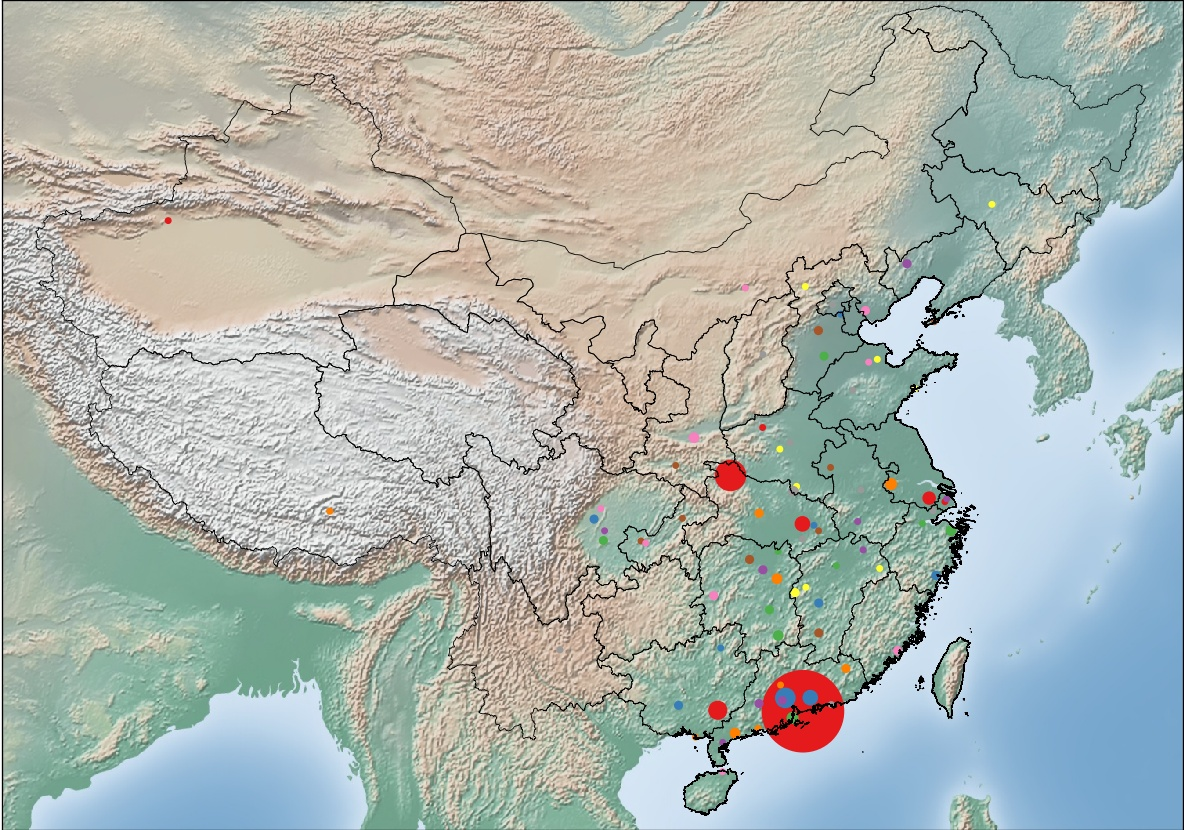# basemap生成地图散点图

1，绘制地图，引用中国和台湾地图，如果你是外国友人，好友主要分布在国外，也可以使用世界地图；
2，统计各个城市好友的数量，映射好友所在城市：城市经纬度坐标形成字典；
3，统计同一个城市好友的数量，映射成城市：数量形成字典；
4，使用range(len(城市的数量))和plt.cm.parameter生成系列颜色的映射并显示在图表上。def drawFriendCityMap():
posDict=getFriendArea()
plt.figure(figsize=(9.87,6.92))
m=Basemap(projection='aea',llcrnrlon=79,llcrnrlat=14,urcrnrlon=138,urcrnrlat=52,lat_0=25,lon_0=110)
m.readshapefile('.\gadm36_CHN_shp\gadm36_CHN_1','states',drawbounds=True)
m.readshapefile('.\gadm36_TWN_shp\gadm36_TWN_0','states',drawbounds=True)
m.shadedrelief()#etopo绘制地形图，shadedrelief是浮雕图，默认是空白轮廓图
fo=open('data.txt','r',encoding='utf-8-sig').readlines()#所有城市坐标的data
wechatPos={}
for k,v in posDict.items():
for j in fo:
a=j.split(',')
if k in a:#如果好友城市在所有城市列表中，将其加入新字典wechpos
wechatPos.update({k:(eval(a),eval(a))})
print(wechatPos)
x1=[]
y1=[]
size=[]
for k,v in wechatPos.items():
x1.append(v)
y1.append(v)
size.append(posDict.get(k))
x,y=m(x1,y1)
m.scatter(x,y,marker='o',s=[v*10 for v in size],c=range(len(wechatPos)),cmap=plt.cm.Set1)#c=range(len(wechatPos)),cmap=plt.cm.viridis
plt.subplots_adjust(left=0, bottom=0, right=1, top=1,hspace=0.1,wspace=0.1)#左下为0右上为1时绘图区全屏
plt.savefig('好友分布图.jpg',dpi=120)